求知 文章 文库 Lib 视频 iPerson 课程 认证 咨询 工具 讲座 Model Center Code

 Python教程 Python快速入门 Python概述 Python环境安装 Python基本语法 Python变量类型 Python基本运算符 Python决策 Python循环 Python数据类型 Python字符串 Python列表 Python元组 Python字典 Python日期时间Date/Time Python函数 Python模块 Python文件I/O Python异常处理 高级教程 Python 3开发网络爬虫(一) Python 3开发网络爬虫(二) Python 3(三): 伪装浏览器 Python 3(四): 登录 Python面向对象 Python正则表达式 Python CGI编程
Python教程
 3281 次浏览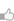110次捐助

Python是一种简单易学，功能强大的编程语言。它包括了高效的高级数据结构和简单而有效的方法，面向对象编程。Python优雅的语法，动态类型，以及它天然的解释能力，使其成为理想的语言，脚本和应用程序快速开发在大多数平台上的许多领域。

Python解释器及其扩展标准库的源码和编译版本可以从Python的Web站点，http://www.python.org/ 所有主要平台可自由查看，并且可以自由发布。该站点上也包含了分配和指针到很多免费的第三方Python模块，程序，工具，以及附加的文档。

Python的解释器很容易扩展新的功能，并在C或C ++（或由C来调用其他语言）实现的数据类型。 Python也很适于作为定制应用的一种扩展语言。

Python环境安装配置

Python数字

Python字符串

Python列表

Python元组

Python字典

1、Python环境安装配置

Python是一种通用的计算机编程语言，跻身排名于世界前八最流行的编程语言。

Repl.it Python 解释器

Ideone.com Python 解释器

Python官方安装指南

PyCharm IDE (桌面IDE)

Atom + script plugin (最小IDE)

 `#!/usr/bin/env pythonprint("Hello World!")print("This is a Python program.")`

（在Python2.x中，打印不必使用中括号，对于 Python3.X 是必需的）

 `Hello World!This is a Python program`

2、Python 数字

 ```#!/usr/bin/pythonx = 3 # an integer stored (in variable x)f = 3.1415926 # a floating real point (in variable f)name = "Python" # a stringbig = 358315791L # long, a very large numberz = complex(2,3) # (2+3i) a complex number. consists of real and imaginary part.print(x)print(f)print(name)print(big)print(z)```

 `33.1415926Python358315791(2+3j)`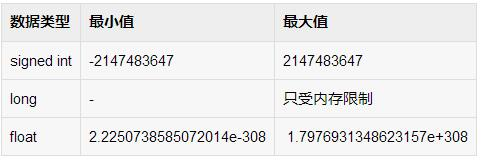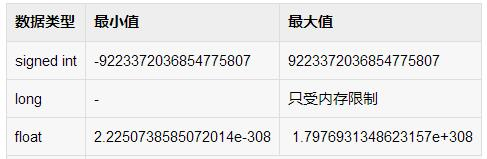`#!/usr/bin/env pythonx = 3y = 8sum = x + yprint(sum)`

 `11`

 `#!/usr/bin/env pythonx = int(raw_input("Enter x:"))y = int(raw_input("Enter y:"))sum = x + yprint(sum)`

 `#!/usr/bin/env pythonx = int(input("Enter x:"))y = int(input("Enter y:"))sum = x + yprint(sum)`

3、Python3 字符串

Python已经在内置支持用于存储和操作文本：字符序列被称为子符串。 要定义字符串应将文本放在引号之间，如果使用单引号（'），双引号（"）或三引号（"""），这并不重要。并无规定最少和最大在字符串中可存储字符的数目。一个空字符串没有文字引号。 例如：

 `s = 'hello's = "hello"s = """hello"""`

 `#!/usr/bin/env pythons = "Hello world" # define the stringprint(s)print(len(s)) # prints the length of the string`

Python索引字符串的字符，每一个索引与一个唯一的字符相关联。例如，在字符串“python”中的字符的索引：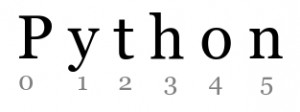Python字符串的索引

 `#!/usr/bin/pythons = "Hello Python"print(s) # prints whole stringprint(s) # prints "H"print(s) # prints "e"`

 `s[ startIndex : pastIndex ]`

startIndex是字符串的开始索引。pastIndex是切片的终点。如果省略了第一个索引，切片将从头开始。如果省略了最后一个索引，切片则到字符串的结尾。 例如：

 `#!/usr/bin/pythons = "Hello Python"print(s[0:2]) # prints "He"print(s[2:4]) # prints "ll"print(s[6:]) # prints "Python"`

 `#!/usr/bin/pythons = "Hello Python"print(s + ' ' + s) # print concatenated string.print(s.replace('Hello','Thanks')) # print a string with a replaced word# convert string to uppercases = s.upper()print(s)# convert to lowercases = s.lower()print(s)`

Python字符串比较，包含和串联

 `#!/usr/bin/pythonsentence = "The cat is brown"q = "cat"if q == sentence: print('strings equal')if q in sentence: print(q + " found in " + sentence)`

 ```#!/usr/bin/env pythonstr1 = "In Python,\nyou can use special characters in strings.\nThese special characters can be..."print(str1)```

 `#!/usr/bin/env pythonstr1 = "The word \"computer\" will be in quotes."print(str1)`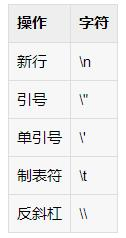、Python 列表

Python有一个数据类型叫列表，被称为 ‘list’. 列表可以包含字符串（文字）和数字。列表使用的是括号定义 []. 要访问数据，使用相同的括号。如字符串，第一元素是。列表用法示例如下：

 `#!/usr/bin/pythonl = [ "Drake", "Derp", "Derek", "Dominique" ]print l # prints all elementsprint l # print first elementprint l # prints second element`

 `['Drake', 'Derp', 'Derek', 'Dominique']DrakeDerp`

 `#!/usr/bin/pythonl = [ "Drake", "Derp", "Derek", "Dominique" ]print l # prints all elementsl.append("Victoria") # add element.print l # print all elementsl.remove("Derp") # remove element.l.remove("Drake") # remove element.print l # print all elements.`

 `['Drake', 'Derp', 'Derek', 'Dominique']['Drake', 'Derp', 'Derek', 'Dominique', 'Victoria']['Derek', 'Dominique', 'Victoria']`

 ```#!/usr/bin/pythonl = [ "Drake", "Derp", "Derek", "Dominique" ]print l # prints all elementsl.sort() # sorts the list in alphabetical orderprint l # prints all elements ```

 `['Drake', 'Derp', 'Derek', 'Dominique']['Derek', 'Derp', 'Dominique', 'Drake']`

 `#!/usr/bin/pythonl = [ "Drake", "Derp", "Derek", "Dominique" ]print l # prints all elementsl.sort() # sorts the list in alphabetical orderl.reverse() # reverse order.print l # prints all elements`

 `['Drake', 'Derp', 'Derek', 'Dominique']['Drake', 'Dominique', 'Derp', 'Derek']`

5、Python 元组

 `tuple = ()`

 `tuple = (3,)`

 `personInfo = ("Diana", 32, "New York")`

 `#!/usr/bin/env pythonpersonInfo = ("Diana", 32, "New York")print(personInfo)print(personInfo)`

 `Diana32`

 `#!/usr/bin/env pythonname,age,country,career = ('Diana',32,'Canada','CompSci')print(country)`

 `Canada`

Python中追加元素到元组

 `#!/usr/bin/env pythonx = (3,4,5,6)x = x + (1,2,3)print(x)`

 `(3, 4, 5, 6, 1, 2, 3)`

 `#!/usr/bin/env pythonlistNumbers = [6,3,7,4]x = tuple(listNumbers)print(x)`

 `#!/usr/bin/env pythonx = (4,5) listNumbers = list(x) print(listNumbers)`

 `#!/usr/bin/env pythonperson = ('Diana','Canada','CompSci')s = ' '.join(person)print(s)`

 `#!/usr/bin/env pythonperson = ('Alison','Victoria','Brenda','Rachel','Trevor')person = tuple(sorted(person))print(person)`

6、Python3 字典

 `#!/usr/bin/pythonwords = {}words["Hello"] = "Bonjour"words["Yes"] = "Oui"words["No"] = "Non"words["Bye"] = "Au Revoir"print words["Hello"]print words["No"]`

 `BonjourNon`

 `#!/usr/bin/pythonsdict = {}dict['Ford'] = "Car"dict['Python'] = "The Python Programming Language"dict = "This sentence is stored here."print dict['Ford']print dict['Python']print dict`

 `CarThe Python Programming LanguageThis sentence is stored here.`

 ```#!/usr/bin/python words = {} words["Hello"] = "Bonjour" words["Yes"] = "Oui" words["No"] = "Non" words["Bye"] = "Au Revoir" print words # print key-pairs. del words["Yes"] # delete a key-pair. print words # print key-pairs. words["Yes"] = "Oui!" # add new key-pair. print words # print key-pairs.```

 ```{'Yes': 'Oui', 'Bye': 'Au Revoir', 'Hello': 'Bonjour', 'No': 'Non'}{'Bye': 'Au Revoir', 'Hello': 'Bonjour', 'No': 'Non'}{'Yes': 'Oui!', 'Bye': 'Au Revoir', 'Hello': 'Bonjour', 'No': 'Non'}```
 您可以捐助，支持我们的公益事业。 1元 10元 50元 认证码：必填
 3281 次浏览110次捐助